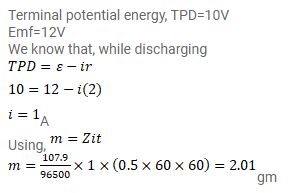# The potential difference across the terminals of a battery of

Question:

The potential difference across the terminals of a battery of emf $12 \mathrm{~V}$ and internal resistance $2 \Omega$ drops to $10 \mathrm{~V}$ when it is connected to a silver voltameter. Find the silver deposited at the cathode in half an hour. Atomic weight of silver is $107.9$ $\mathrm{g} /$ mole.

Solution: